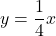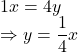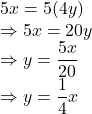## Kelly and Greta are asked to write an equation for the scenario below. “One person was able to plant 4 trees in the same amount of time that

Question

Kelly and Greta are asked to write an equation for the scenario below. “One person was able to plant 4 trees in the same amount of time that 5 people working together were able to plant 20 trees.” The girls agree that x represents the number of people working and y represents the number of trees planted. Kelly wrote the equation y = one-fourth x, and Greta wrote the equation y = 4 x. Which person is correct, and why? Kelly is correct; each person plants One-fourth more trees than the person before them. Kelly is correct; 1 = one-fourth times 4 and 5 = one-fourth times 20. Greta is correct; each person plants 4 more trees than the person before them. Greta is correct; 4 = 4 times 1 and 20 = 4 times 5.

in progress 0
2 months 2021-07-28T08:26:06+00:00 2 Answers 1 views 0

d is correct non edge 2021

Step-by-step explanation:Kelly is correct.

Step-by-step explanation:

One person is able to plant 4 trees

Let x be the number of people

y be the number of trees

The situation is represented by5 people are able to plant 20 treesSo, the situation is represented byHence, Kelly is correct.